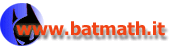www.batmath.it

### Step functions

Step functions are functions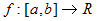, that are constant on sub-intervals of [a,b]; they are also called piecewise constant functions. In order to give a precise definition of this kind of functions it's useful to introduce the concept of dissection (or subdivision) of an interval of real numbers.

A dissection of an interval [a,b] of real numbers is a set of n+1 points such that: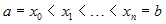. We'll use the traditional set notation for dissections: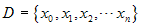.

Given a dissection of [a,b], the interval [a,b] can be written as, that is as a finite union of subintervals: these subintervals are generated by the dissection.

#### Step functions

A functionis called a step function on [a,b] if there exists a dissection D of [a,b] and real numbers
c
1, c2, ... , cn, such that, for every k = 1, 2, ... ,n,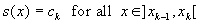.

Observe that we have imposed no condition on s(xk), except that they are real valued. This is in view of the fact that the Riemann integral is unchanged if we alter the value of the function in a finite number of points.

#### Examples

• The function f(x)=signum(x), in the interval [-1,1] is a step function.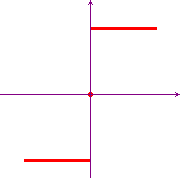• The function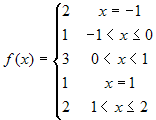is a step function.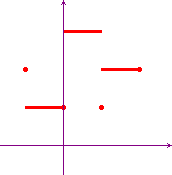• An important example of step function is the following:  f(x)=[x] = "the greatest integer ≤ x". This is a step function on every interval [a,b] and is called the characteristic function or the integral part function.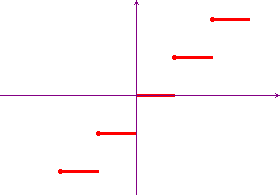Given a dissection D of [a,b], if you add some points to the set D you obtain a new dissection D'. Clearly D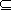D'. D' is called a refinement of D. If f is a step function and D is a dissection of [a,b], the function is constant also in the subintervals generated by every dissection D' such that DD'. This means that the dissection is not uniquely determined by the step function. However this fact is unimportant for our theory.

#### Sums and products of step functions

Given two step functions, s and t, and the corresponding two dissections D and E of their common domain [a,b], it's a useful exercise to prove that the two functions s+t and s·t are step functions and a possible dissection of [a,b] is simply DE.

#### Examples

• The function f(x) = [x] + signum(x) in [-1,2]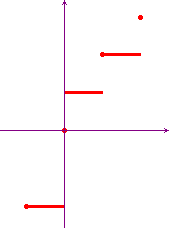• The function f(x) = [x] · signum(x) in [-1,2].first published on january 07 2003 - last updated on september 01 2003